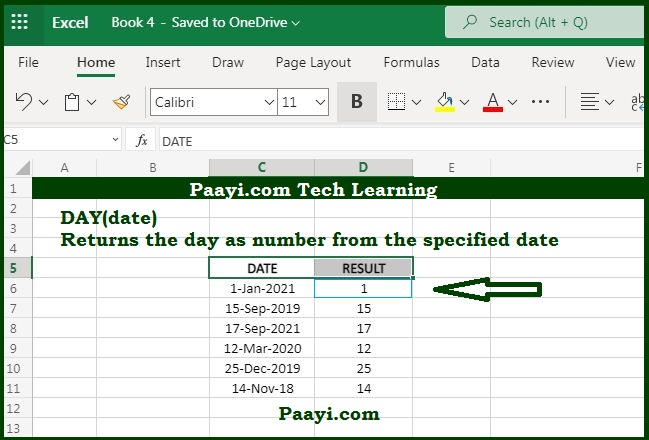# Learn How to Use Microsoft Excel DAY Function

Written by | 0 Comments | 769 Views

In this article, you will learn how to use the Microsoft Excel DAY function and its prime function in Microsoft Excel. You will also get to know the Microsoft Excel DAY function return value and syntax with the help of some examples.

## Microsoft Excel Day Function

The DAY function's prime purpose is to get a day as a number (1-31) from the date. So, with the Microsoft Excel DAY function, you can return the day of the month in numerals ranges between 1 to 31 from the provided date. With the DAY function, you can extract the day number from the date entered into the cell. Besides that, you can use the DAY function to extract and feed a day value into another function, similar to the DATE function.

### DAY Function Return Value

It will return a number ranged between "1 to 31" to represent a day component in a date.

### DAY Function Syntax

= DAY(date)

Where:

• day: It is a valid Excel date.

### How to Use Microsoft Excel DAY Function?As we know, the DAY function will return the day value in numbers between "1 to 31" from a given date. Let us understand this by the example given below:

• = DAY(A1)

If the date in cell A1 is 15 January 2020, it will return the number 15.

The DAY function can also be used to extract from a date into a cell. Besides, you can use the DAY function to extract a feed value into another function, similar to the DATE function. Let's understand this by the example given below:

• = DAY(2020,MONTH(A1),DAY(A1))

Using this you can change the year in the date, but the month and day will be as it is.